# COMMON CLUSTERING ALGORITHMS (PART 1)

## Affinity Propagation

##### Similarity Score & Similarity Matrix

The similarity score s(i, j) is calculated using -||xi-xj||² For s(i, i), we could fill them in with the lowest value among all cells.

##### Responsibility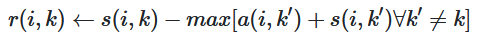In this formula, i refers to the row and k the column of the associated matrix. (All values for a is set to zero initially)

##### Availability

For elements in the diagonal: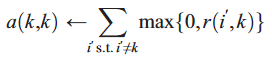For other elements: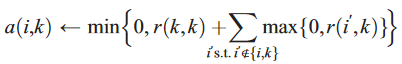Similarly, i refers to the row and k the column of the associated matrix.

##### Criteria

The criteria score equals the sum of responsibility and availability:

Rows that share the same exemplar are in the same cluster.

## Agglomerative Clustering

It is also called bottom-up clustering.

##### Calculate Distance

A common way to calculate distance between two points is Euclidean Distance: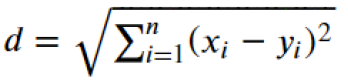After calculating all pair-wise distances, we merge the smallest non-zero distance in the matrix as a new node.

• In single linkage clustering, the similarity of two clusters is the similarity of their most similar members, which is the minimum distance between cluster data points.
• In complete-linkage clustering , the similarity of two clusters is the similarity of their most dissimilar members, which is the maximum distance between data points.
• In average linkage clustering, the distance between two clusters is defined as the average of distances between all pairs of data points.
• Ward´s Method seeks to choose the successive clustering steps so as to minimize the increase in ESS (Error Sum of Squares) at each step.

Upon calculating the pair-wise distances, we could repeat merging and calculating the distances until all data points are merged into one cluster.

##### Finding the Number of Clusters

After we get our dendrogram, we still need to choose a number of clusters since the dendrogram only demonstrates the hierarchy. Usually we could visualize the dendrogram and cut at the longest branch, or cut at branches that are longer than a specific value, but there are also some dynamic cutting methods.

## BIRCH

##### Clustering Feature

A Clustering Feature (CF) is defined as a triplet (N, LS, SS), where

• N is the number of data points in the CF
• LS is the linear sum of data points in the CF
• SS is the square sum of data points in the CF

For example, assume there are 5 data points (3, 4), (2, 6), (4, 5), (4, 7), (3, 8), then the corresponding CF equals (5, (16, 30), (54, 190))

When adding two CFs, say CF1 = (N1, LS1, SS1) and CF2 = (N2, LS2, SS2), the sum is

##### Phase 1: Building a CF Tree

Before introduce how to build a CF Tree, there are some key concepts:

• Threshold (T): The maximum number of data points that a leaf node can hold
• Branching Factor (B): The maximum number of CF sub-clusters that a non-leaf node can hold
• Centroid (X0)
•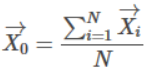• Radius (R): The average distance from member points to the centroid
•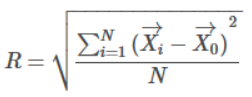• Diameter (D): The average pairwise distance within a cluster
•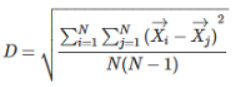To form a CF tree, we have following steps: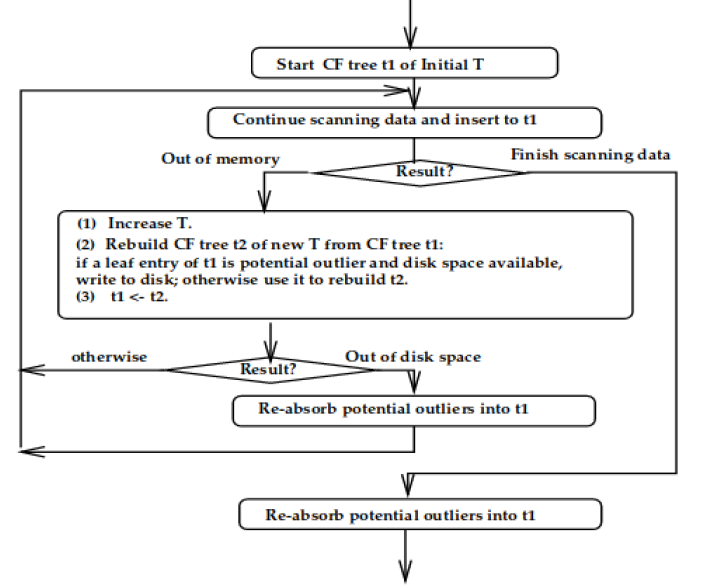1. Start with initial values of T and B, we keep scanning through data points. Each time when a new data point is added, we find the closest leaf node using a chosen distance criteria (e.g. Euclidean distance or Manhattan distance or something else)
2. Upon finding the closest leaf node, we check if adding this data point to the node will violating the threshold condition
• If it does not violate, update the CF entry
• Otherwise, check if a new entry could be added to the leaf
• If there’s space for this new entry, we are done
• Otherwise, split the leaf node by choosing the farthest pair of entries as seeds and redistributing the remaining entries based on the distance criteria
3. After inserting the data point, we update the CF information for each non-leaf node. In the event of a split, we must insert a new non-leaf node into the parent node and have it point to the newly formed leaf. If the parent has space for new nodes, we only need to update CF vectors, but generally we may need to split the non-leaf parent nodes as well
##### Phase 2: Condense the Tree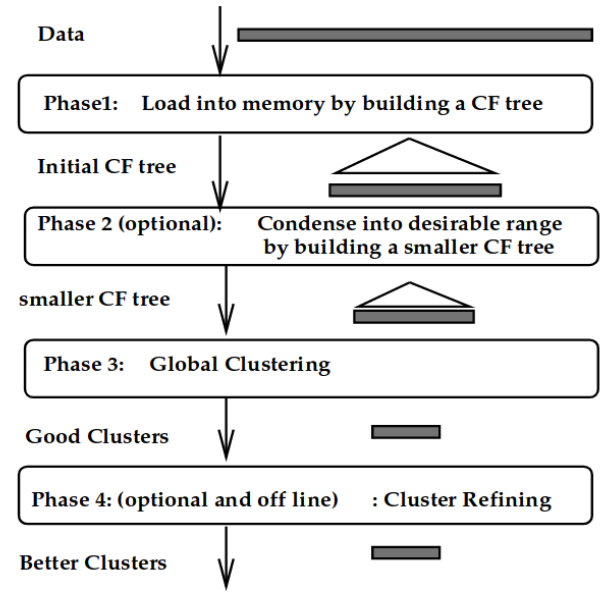Although it is optional, phase 2 may work as a cushion to rebuild a smaller CF tree, removing more outliers and grouping crowded sub-clusters into larger ones.

##### Phase 3: Global Clustering

Due to skewed input order and splitting triggered by page size, we may use a global or semi-global algorithm to cluster all leaf nodes. Almost any existing clustering algorithms could be adapted to work with sub-clusters. For sub-clusters, we can:

1. Use the centroid as the representative of each cluster, treat each sub-cluster as a single point and use an existing algorithm without modification
2. Treat a sub-cluster of n data points as its centroid repeating n times and modifying an existing algorithm slightly to take the counting information into account
3. Directly apply an existing algorithm to sub-clusters using CF vectors
##### Phase 4: Cluster Refining

Phase 4 utilizes centroids of clusters produced by phase 3, and redistributes data points to the closest centroids. It guarantees all copies of a given data point go to the same cluster, correcting the inaccuracy caused by the algorithm. Besides, phase 4 could also discard outliers.